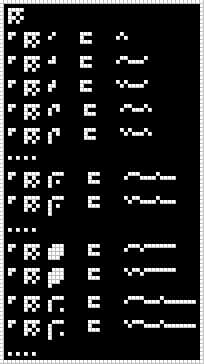# #13. Modulate¶

Note

Following documentation is a cooperative result combined from our Discord chat and numerous pull requests. Thanks to everyone who helped!

## Image¶

This image was produced from the thirteenth radio transmission using previously contributed code.This partly annotated version of the image was made using code from message #3.## Interpretation¶

The operator defined in this message, mod, is for converting numbers from a grid form into a linear-encoded form. The linear encoding appears to be a type of Variable-length encoding, with the following form:

• Bits 0..1 define a positive or negative number (and signal width) via a high/low or low/high signal change:

• 01: positive number

• 10: negative number

• Bits 2..(n+2) define the width of the following binary-encoded number via a unary-encoded number of length n composed of high signals ending with a low signal. The number width (in bits) is four times the unary encoding (i.e. 4 * n):

• 0: 0 [i.e. the number zero]

• 10: 4-bit number [i.e. 1-15]

• 110: 8-bit number [i.e. 1-255]

• 1110: 12-bit number [i.e. 1-4095]

• The remaining bits, i.e. (n + 3)..(n + 3 + 4*n - 1), determine the number itself, in most-significant-bit first binary notation. Using the examples from this message:

• 0001: 1

• 00010000: 16

• 000100000000: 256

With this encoding, the number zero only requires three bits (i.e. 010), but arbitrarily large numbers can also be represented.

## Decoded¶

mod
ap mod 0   =   
ap mod 1   =   
ap mod -1   =   [-1]
ap mod 2   =   
ap mod -2   =   [-2]
...
ap mod 16   =   
ap mod -16   =   [-16]
...
ap mod 255   =   
ap mod -255   =   [-255]
ap mod 256   =   
ap mod -256   =   [-256]
...


## Code¶

The Haskell code has been revised to decode new glyphs.

Example output: